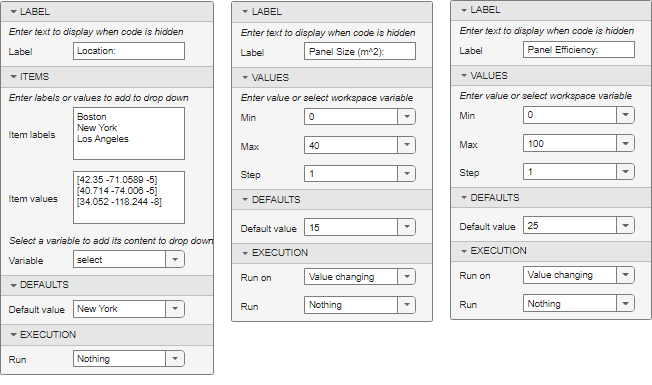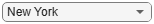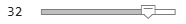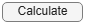# Create an Interactive Form Using the Live Editor

In the Live Editor, you can create interactive forms or simple apps to perform small, repeatable tasks. When creating these forms or apps, you can use interactive controls to prompt for input and perform the tasks. To show only the formatted text, controls, and results to the user, hide the code.

This example shows how to create a basic interactive form in the Live Editor that completes a calculation based on input provided by a user. The form uses a drop-down list and numeric sliders to prompt the user for input, and then uses a button to run a calculation and plot a graph using the provided input.

To view and interact with the Solar Panel Output Estimator form, open this example in MATLAB®.### Create the Form

You can open a copy of the Solar Panel Output Estimator form by opening this example in MATLAB. To recreate the form yourself, create a live script named `SolarPanelEstimatorForm.mlx`. Then, add the descriptive text and code, configure the controls, and hide the code.

Copy the descriptive text and code in the Code for Solar Panel Output Estimator Form section to calculate the output of a set of solar panels based on the location, size, and efficiency of the panels.

#### Configure the Controls

The form uses a drop-down list and numeric sliders to prompt the user for input and a button to run a calculation and plot a graph using the provided input.

When you copy the code, the controls are replaced with their current value. To add the controls back into the code, replace the value of the location variable with a drop-down list and the values of the `pSize` and `eff` variables with numeric sliders. Then, configure the controls by right-clicking them, selecting Configure Control, and specifying the control options as follows:

• location drop-down list — Set the Label to `Location:` and the Item labels and Item values to a set of locations and their corresponding coordinates. Set the Run execution option to `Nothing`.

• pSize slider — Set the Label to `Panel Size (m^2):` and the Min and Max values to `0` and `40`, respectively. Set the Run execution option to `Nothing`.

• eff slider — Set the Label to `Panel Efficiency:` and the Min and Max values to `0` and `100`, respectively. Set the Run execution option to `Nothing`.To add the button back into the code, at the end of the code, insert a button. Then, configure the button by right-clicking it and selecting Configure Control. Set the Label to `Calculate` and the Run execution option to `Current section`. When a user presses the button, the code in the current section runs, updating the calculation based on the current values of the drop-down list and sliders.

#### Hide the Code

To view the example as a form, with the code hidden and only the controls and results visible, go to the View tab and click Hide Code. Users can now interact with the form by choosing from the drop-down list, adjusting the sliders, and clicking the button to view the results. The Live Editor calculates solar panel output estimates based on user-provided inputs.

### Code for Solar Panel Output Estimator Form

This section provides the complete contents of the SolarPanelEstimatorForm.mlx live script file, including the descriptive text, code, and sample results.

#### Solar Panel Output Estimator

Specify the location of your panels.

```% Calculate Solar Time location =[40.714 -74.006 -5]; lambda = location(2); % Longitude phi = location(1); % Latitude UTCoff = location(3); % UTC offset if(UTCoff < 0) TZ = "UTC" + num2str(UTCoff); else TZ = "UTC+" + num2str(UTCoff); end january1 = datetime(2016,1,1,"TimeZone",TZ); % January 1st localYear = 2018; localMonth = 6; localDay = 1; localHour = 12; localTime = datetime(localYear,localMonth,localDay,localHour,0,0,"TimeZone",TZ); d = caldays(between(january1,localTime,"Day")); % Day of year solarCorr = solarCorrection(d,lambda,UTCoff); % Correction to local time solarTime = localTime + minutes(solarCorr); % Calculate Solar Declination and Elevation delta = asind(sind(23.45)*sind(360*(d - 81)/365)); % Declination omega = 15*(solarTime.Hour + solarTime.Minute/60 - 12); % Hour angle alpha = asind(sind(delta)*sind(phi) + ... % Elevation cosd(delta)*cosd(phi)*cosd(omega)); % Calculate Air Mass and Solar Radiation AM = 1/(cosd(90-alpha) + 0.50572*(6.07955+alpha)^-1.6354); sRad = 1.353*0.7^(AM^0.678); % kW/m^2 % Calculate Solar Radiation on Fixed Panels gamma = acosd((sind(delta)*cosd(phi) - cosd(delta)*sind(phi)*cosd(omega))/cosd(alpha)); if (hour(solarTime) >= 12) && (omega >= 0) gamma = 360 - gamma; end beta = 180; % Panel azimuth tau = 35; % Panel tilt pRad = sRad*max(0,(cosd(alpha)*sind(tau)*cosd(beta-gamma) + sind(alpha)*cosd(tau))); ```

Specify the panel size and efficiency value.

```% Calculate Panel Size and Efficiency pSize =32; % Panel size in m^2 eff =25; % Panel efficiency pElec = eff*pSize*pRad; % Panel electric output in kW```

#### Results:

`disp("Expected electrical output = " + num2str(pElec) + " kW")`
```Expected electrical output = 717.5021 kW ```
```% Calculate Power Generation Over Time isFixed = 1; date = datetime(localYear,localMonth,localDay,"TimeZone",TZ); [times,sRad,pRad] = hourlyPanelRadiation(date,lambda,phi,UTCoff,tau,beta,isFixed); plot(times,sRad,times,pRad) title("Solar and Panel Radiation for " + string(date,"mmmm dd yyyy")) xlabel("Hour of Day"); ylabel("Radiation, kW/m^2") legend("Available Solar Radiation","Solar Radiation on Panel","Location","South")```